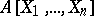# Hilbert theorem

(diff) ← Older revision | Latest revision (diff) | Newer revision → (diff)
Hilbert's basis theorem. Ifis a commutative Noetherian ring andis the ring of polynomials inwith coefficients in, thenis also a Noetherian ring. In particular, in a ring of polynomials in a finite number of variables over a field or over a ring of integers any ideal is generated by a finite number of elements (has a finite basis). This is the form in which the theorem was demonstrated by D. Hilbert ; it was used as auxiliary theorem in the proof of Hilbert's theorem on invariants (see below, 8). Subsequently, Hilbert's basis theorem was extensively used in commutative algebra.